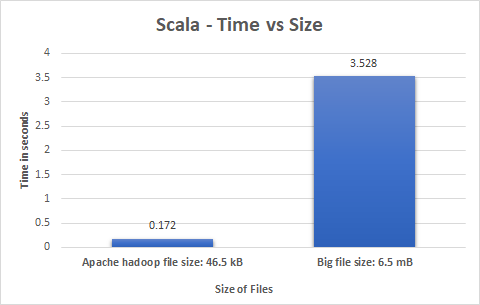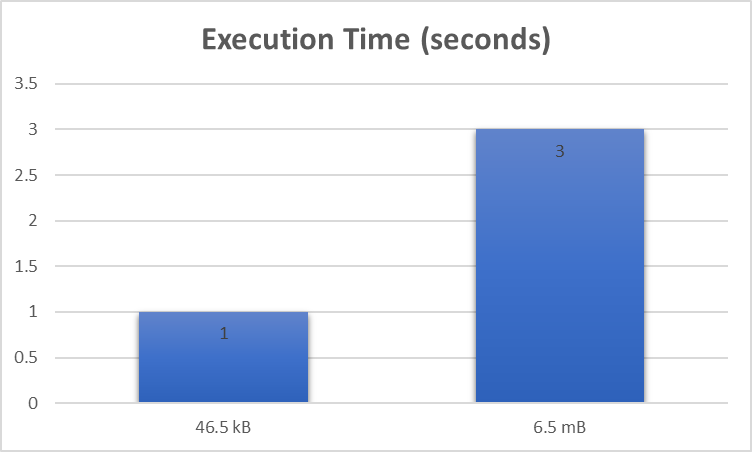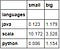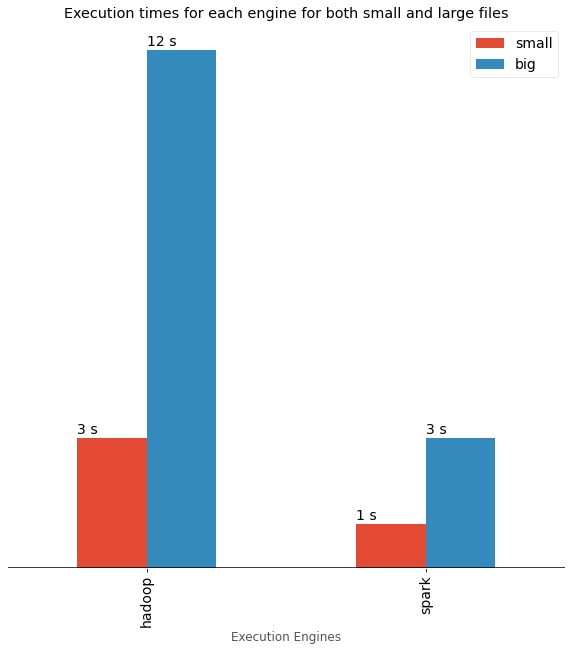# Data Analysis

## Python

`# Reading filefile=open("../Input-Files/apache-hadoop-wiki.txt","r",encoding="utf-8")`
`# Initializing Dictionarydict = {}# counting number of times each word comes up in list of words (in dictionary)for word in file.read().split():    dict[word] = dict.get(word, 0) + 1file.close()`
`#write the filefw = open("small-result-python.txt","w",encoding="utf-8")fw.write(str(dict))fw.close()`
`{'Apache': 79, 'Hadoop': 201, 'From': 3, 'Wikipedia,': 1, 'the': 211, 'free': 1, 'encyclopedia': 1, 'Jump': 2, 'to': 122 }`
`import timestart = time.time()# Reading filefile=open("../Input-Files/apache-hadoop-wiki.txt","r",encoding="utf-8")# Initializing Dictionarydict = {}# counting number of times each word comes up in list of words (in dictionary)for word in file.read().split():     dict[word] = dict.get(word, 0) + 1file.close()#write the filefw = open("small-result-python.txt","w",encoding="utf-8")fw.write(str(dict))fw.close()end = time.time() print("Execution time :", end - start)`(Left) Bar chart depicting processing times for Python | (Middle) Bar chart depicting processing times for Java | (Right) Bar chart depicting processing times for Scala | Images by Author.

## Execution Engines(left) Graph depicting processing time taken by Hadoop | (right) Graph depicting processing time taken by Spark | Images by Author

# Comparative Analytics

`import pandas as pdimport matplotlib as mplimport matplotlib.pyplot as pltmpl.style.use('ggplot')`
`# initialize list of languages data_lan = [['java', 0.123, 1.179 ], ['scala', 10.172, 3.528]]   # Create the pandas DataFrame df_languages = pd.DataFrame(data_lan, columns = ['languages', 'small','big'])   # print dataframe. df_languages`
`# Appending Data, Use this method in the future when trying to add more languagesdf_languages = df_languages.append({'languages':'python','small':0.006, 'big':1.154}, ignore_index=True)df_languages# Set the languages as index for our x axisdf_languages.set_index('languages', inplace=True)df_languages`Dataset with clear X & Y axis demarcations | Image by Author
`ax = df_languages.plot(kind='bar',                      figsize = (10,10)                      )plt.xlabel('Languages')plt.title('Execution times for each language for both small and large files')ax.set_facecolor('white')ax.tick_params(axis='x', colors='black', labelsize=14)ax.axhline(0, color='black')ax.legend(facecolor='white',fontsize=14)ax.tick_params(top=False, left=False, right=False, labelleft=False)for p in ax.patches:   #display the percentages above the bars    width, height = p.get_width(), p.get_height()    x, y = p.get_xy()     ax.annotate('{} s'.format(height), (x, y + height + 0.1),fontsize=14)plt.show()`

# Conclusion(left) Graph comparing & benchmarking the file processing time for Programming languages | (right) Graph comparing & benchmarking the file processing time for Execution Engines | Images by Author

# References

## Geek Culture

A new tech publication by Start it up (https://medium.com/swlh).

Written by

## Thomas George Thomas

Data Analytics Engineering Graduate Student at Northeastern. Ex Senior Data Engineer & IBM Certified Data Scientist. https://thomasgeorgethomas.ml## Geek Culture

A new tech publication by Start it up (https://medium.com/swlh).